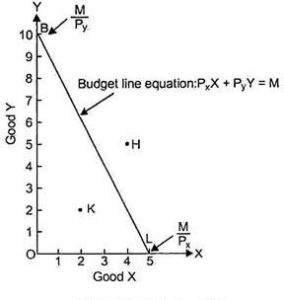# Consumer’s Budget: Budget Set, Slope of a Budget Line, Examples

The compilation of these Consumer Behaviour Notes makes students exam preparation simpler and organised.

## Consumer’s Budget

Have you ever prepared a monthly budget? How do you decide how much of a good to buy? And do you ever change your preferences? This can be understood by the economic concept of consumer budget. The concepts of the budget line, budget set, and slope of the budget are explored here. Read on.

### Introduction of Variables and Basic Concepts

The concept of consumer preferences is integral to understanding consumer budgets. In microeconomics, we understand consumer preferences using two goods, say 1 and 2. Let their prices be P1 and P2 and their quantities consumed be X1 and X2. To understand the budget, we must introduce the idea of the money income of the consumer. Let that be denoted by ‘M’.

Consumer preferences are denoted by quantities of the two goods consumers are willing to purchase depicted using ‘bundles’. A bundle is a combination of good 1 and 2, written as (good 1, good 2). Thus, a bundle written as (1, 2) means 1 unit of good 1 and 2 units of good 2. Similarly, (3, 7); (8, 4) are also bundles with different quantities of good 1 and 2.

### Important Terminologies

Monotonic Preferences
A rational consumer will always prefer more goods to less. Thus, if there is one bundle with more of both goods than the second bundle, the consumer will always prefer to consume the first bundle. Even if one bundle contains the same of the first good but more of the other good than the second bundle, a consumer will prefer the first bundle. This is called the concept of monotonic preferences.

Example 1:
Bundle A: (4, 9)
Bundle B: (3, 6)

The consumer prefers Bundle A to Bundle B.

Example 2:
Bundle A: (4, 9)
Bundle B: (4, 5)

The consumer prefers Bundle A to Bundle B.

### Consumer Budget

A consumer budget is the real purchasing power with which he can purchase quantities of two goods, given their prices.

Budget Set
There are two factors that affect a consumer’s choice of quantities to purchase between two goods: prices and money income. Price times quantity for each good gives us the expenditure incurred on purchasing a good. In our analysis, there are two goods. So, the expenditure on both goods must be less than or equal to the money income of the consumer. This is the budget constraint faced by a consumer. It is depicted as:

P1.X1 + P2.X2 ⇐ M

All those bundles that satisfy this criterion are set to form a part of what is called the budget set. The consumer can choose to consume out of any of these bundles.

Budget Line
In the consumer budget, the graphical representation of all such bundles which cost the consumer exactly his money income is called the budget line. The equation of the budget line is, therefore:

P1.X1 + P2.X2 = M

We measure the quantity of good 1 on the horizontal axis and that of good 2 on the vertical axis. We can depict the budget line by calculating the horizontal and vertical intercept. The intercepts are the maximum of each good the consumer can afford to buy.

So, horizontal intercept = M/P1 (the consumer buys only good 1, X2 = 0)
And, vertical intercept = M/P2 (the consumer buys only good 2, X1 = 0)The figure assumes good 1 to be ‘X’ and good 2 to be ‘Y’. Note that a bundle such as ‘K’ forms a part of the budget set and costs less than the total money income. A bundle such as ‘H’ on the other hand lies outside the budget line and is hence not affordable.

Slope of the Budget Line
Why does the budget line slope downward? We must understand that the quantities of goods 1 and 2 are limited (as in the real world) and the consumer has a fixed money income. So, to have more of good 1, he has to ‘give up’ some amount of good 2. This negative relation between consumption quantities of two goods causes the budget line to slope downwards.

The slope of the budget line is the amount of good 2 given up to have one more unit of good 1. The price of one unit of good 1 is P1. To have one more unit of good 1, therefore, consumption of good 2 must be reduced by P1 amount. Now, with the P2 amount, one unit of good 2 could have been bought. So, with the P1 amount, of good 2 could have been bought. Thus, the consumer must give up units of good 2 to obtain one extra unit of good 1, i.e. the slope of the budget line is.

Example:

Question:
What is a budget set? When can it change?